# DAV Class 5 Maths Chapter 7 Worksheet 12 Solutions

The DAV Maths Book Class 5 Solutions and DAV Class 5 Maths Chapter 7 Worksheet 12 Solutions of Multiplication and Division of Decimal Numbers offer comprehensive answers to textbook questions.

## DAV Class 5 Maths Ch 7 Worksheet 12 Solutions

Question 1.
Complete the following:
(a)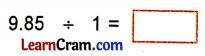Solution:
9.85 ÷ 1 = 9.85

(b)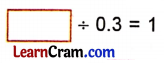Solution:
0.3 ÷ 0.3 = 1(c)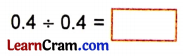Solution:
0.4 ÷ 0.4 = 1

(d)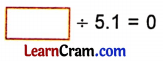Solution:
0 ÷ 5.1 = 0

(e)Solution:
0 ÷ 19.1 = 0(f)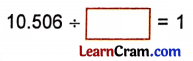Solution:
10.506 ÷ 10.506 = 1

(g)Solution:
16.032 ÷ 16.032 = 1

(h)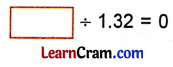Solution:
0 ÷ 1.32 = 0

DAV Class 5 Maths Chapter 7 Worksheet 12 Notes

A decimal number divided by one is the decimal number itself.
Example:
(a). 8.9 ÷ 1 = 8.9
(b) 0.036 ÷ 1 = 0.036

Zero divided by any decimal number is zero.
0 ÷ 4.6 = $$\frac{0}{4.6}$$
= $$\frac{0 \times 10}{4.6 \times 10}$$
= $$\frac{0}{46}$$
= 0A decimal number divided by itself is one
Example:
0.2 ÷ 0.2 = $$\frac{0.2}{0.2}$$
= $$\frac{0.2 \times 10}{0.2 \times 10}$$
= 1

0 ÷ 0.07 = $$\frac{0}{0.07}$$
= $$\frac{0 \times 100}{0.07 \times 100}$$
= $$\frac{0}{7}$$
= 0